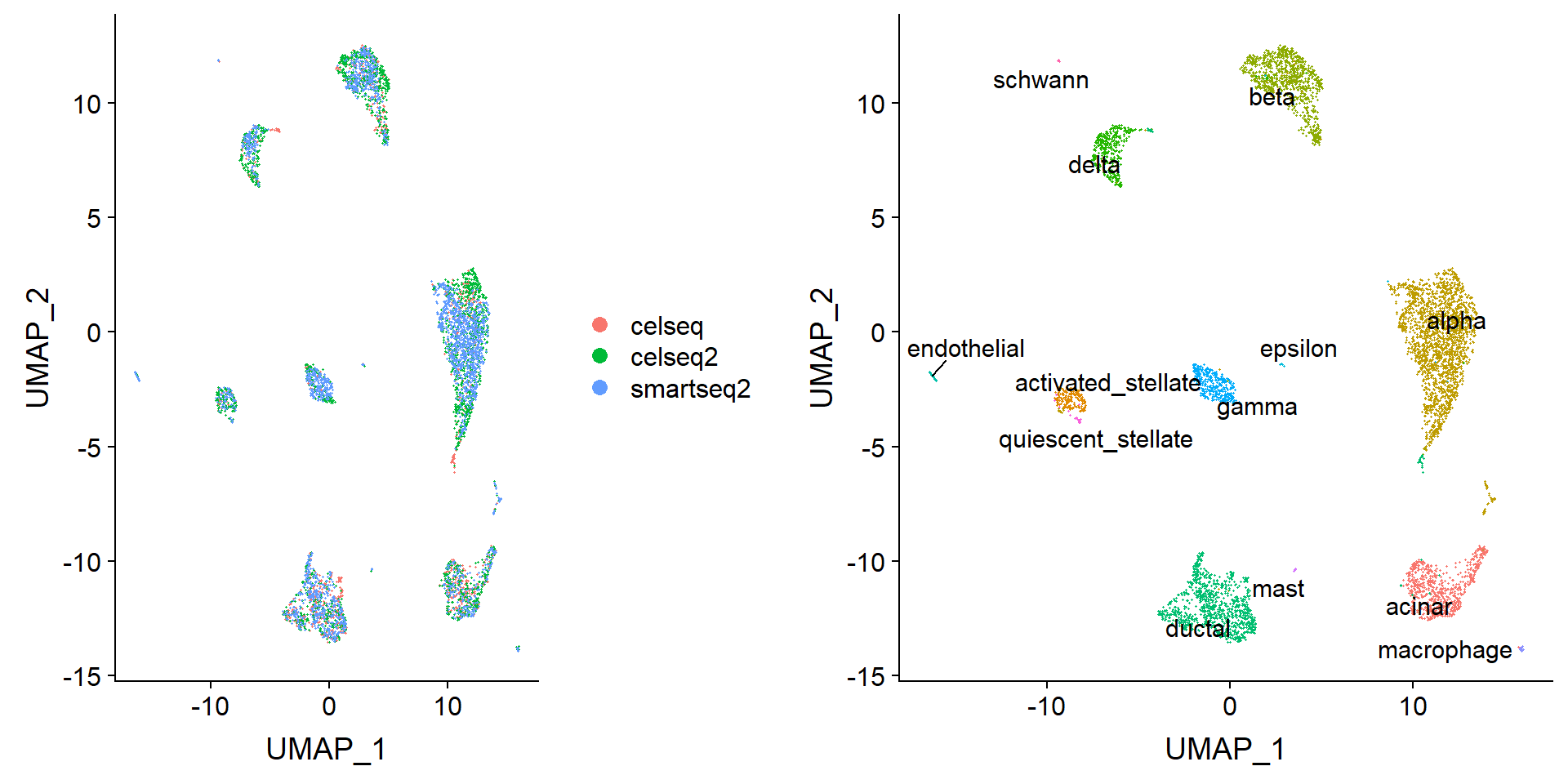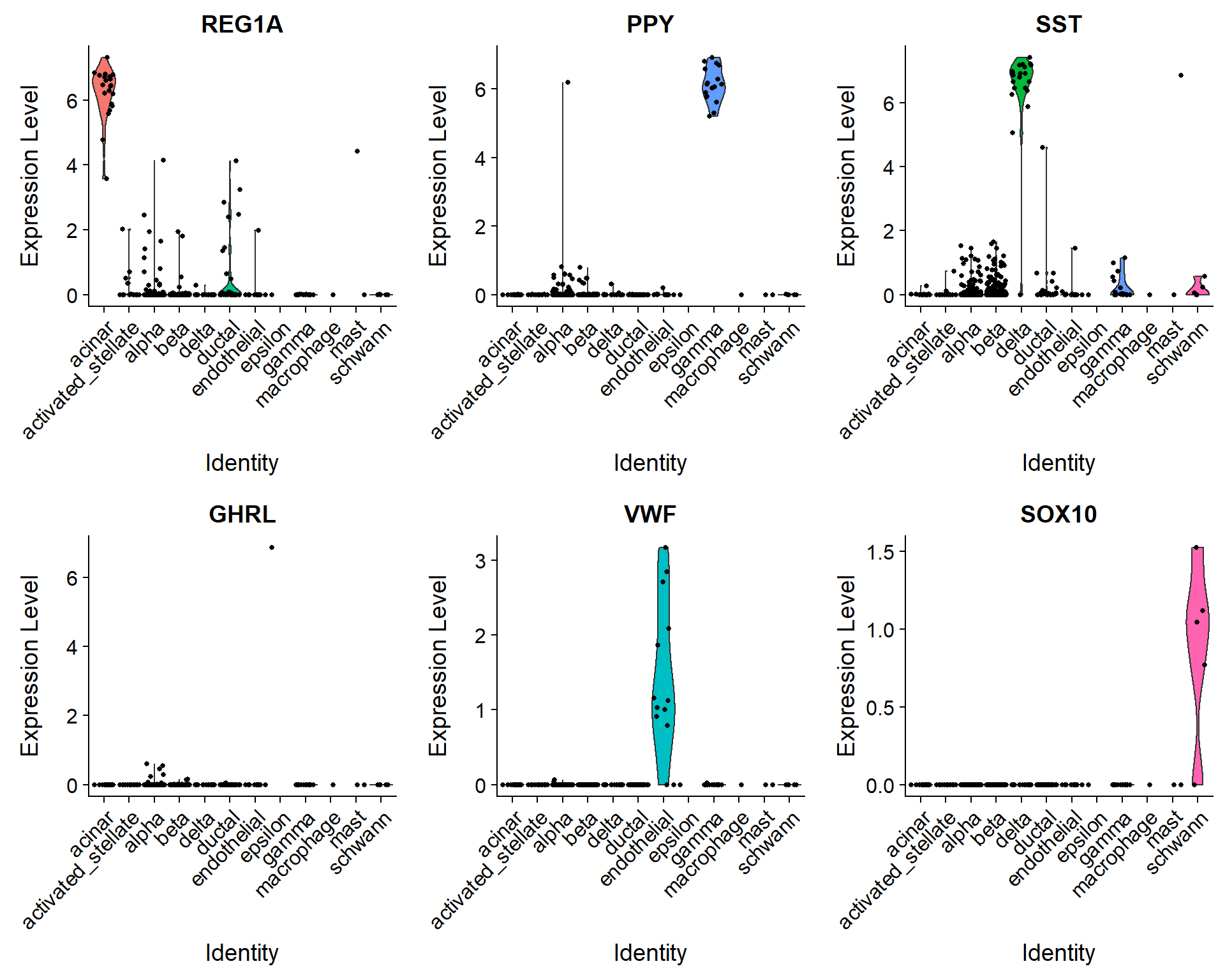# Seurat: Integration and Label Transfer

#### Presented by: Tim Stuart (@timoast) and Andrew Butler (@andrewwbutler)

April 25 2019

Slides

In this example workflow, we demonstrate two new methods we recently introduced in our preprint, Comprehensive Integration of Single Cell Data:

• Assembly of multiple distinct scRNA-seq datasets into an integrated reference
• Transfer of cell type labels from a reference dataset onto a new query dataset

For the purposes of this example, we’ve chosen human pancreatic islet cell datasets produced across four technologies, CelSeq (GSE81076) CelSeq2 (GSE85241), Fluidigm C1 (GSE86469), and SMART-Seq2 (E-MTAB-5061). We provide a combined raw data matrix and associated metadata file here to get started.
The code for the new methodology is implemented in Seurat v3. You can download and install from CRAN with `install.packages`.

``install.packages("Seurat")``

In addition to new methods, Seurat v3 includes a number of improvements aiming to improve the Seurat object and user interaction. To help users familiarize themselves with these changes, we put together a command cheat sheet for common tasks. We are preparing a full release with updated vignettes, tutorials, and documentation in the near future.

### Dataset preprocessing

Load in expression matrix and metadata. The metadata file contains the technology (`tech` column) and cell type annotations (`cell type` column) for each cell in the four datasets.

``library(Seurat)``
``## Warning: package 'Seurat' was built under R version 3.5.3``
``````pancreas.data <- readRDS(file = "../data/pancreas_expression_matrix.rds")

To construct a reference, we will identify ‘anchors’ between the individual datasets. First, we split the combined object into a list, with each dataset as an element.

``````pancreas <- CreateSeuratObject(counts = pancreas.data, meta.data = metadata)
pancreas.list <- SplitObject(object = pancreas, split.by = "tech")``````

Prior to finding anchors, we perform standard preprocessing (log-normalization), and identify variable features individually for each. Note that Seurat v3 implements an improved method for variable feature selection based on a variance stabilizing transformation (`"vst"`)

``````for (i in 1:length(x = pancreas.list)) {
pancreas.list[[i]] <- NormalizeData(object = pancreas.list[[i]], verbose = FALSE)
pancreas.list[[i]] <- FindVariableFeatures(object = pancreas.list[[i]], selection.method = "vst",
nfeatures = 2000, verbose = FALSE)
}``````

### Integration of 3 pancreatic islet cell datasets

Next, we identify anchors using the `FindIntegrationAnchors` function, which takes a list of Seurat objects as input. Here, we integrate three of the objects into a reference (we will use the fourth later in this vignette)

• We use all default parameters here for identifying anchors, including the ‘dimensionality’ of the dataset (30; feel free to try varying this parameter over a broad range, for example between 10 and 50).
``````reference.list <- pancreas.list[c("celseq", "celseq2", "smartseq2")]
pancreas.anchors <- FindIntegrationAnchors(object.list = reference.list, dims = 1:30)``````

We then pass these anchors to the `IntegrateData` function, which returns a Seurat object.

• The returned object will contain a new `Assay`, which holds an integrated (or ‘batch-corrected’) expression matrix for all cells, enabling them to be jointly analyzed.
``pancreas.integrated <- IntegrateData(anchorset = pancreas.anchors, dims = 1:30)``

After running `IntegrateData`, the `Seurat` object will contain a new `Assay` with the integrated expression matrix. Note that the original (uncorrected values) are still stored in the object in the “RNA” assay, so you can switch back and forth.
We can then use this new integrated matrix for downstream analysis and visualization. Here we scale the integrated data, run PCA, and visualize the results with UMAP. The integrated datasets cluster by cell type, instead of by technology.

``library(ggplot2)``
``## Warning: package 'ggplot2' was built under R version 3.5.3``
``library(cowplot)``
``## Warning: package 'cowplot' was built under R version 3.5.3``
``````# switch to integrated assay. The variable features of this assay are automatically set during
# IntegrateData
DefaultAssay(object = pancreas.integrated) <- "integrated"

# Run the standard workflow for visualization and clustering
pancreas.integrated <- ScaleData(object = pancreas.integrated, verbose = FALSE)
pancreas.integrated <- RunPCA(object = pancreas.integrated, npcs = 30, verbose = FALSE)
pancreas.integrated <- RunUMAP(object = pancreas.integrated, reduction = "pca", dims = 1:30)
p1 <- DimPlot(object = pancreas.integrated, reduction = "umap", group.by = "tech")
p2 <- DimPlot(object = pancreas.integrated, reduction = "umap", group.by = "celltype", label = TRUE,
repel = TRUE) + NoLegend()
plot_grid(p1, p2)````````saveRDS(object = pancreas.integrated, file = "../output/pancreas_integrated.rds")``
``````plot <- DimPlot(object = pancreas.integrated, reduction = "umap", label = TRUE, label.size = 4.5) +
xlab("UMAP 1") + ylab("UMAP 2") + theme(axis.title = element_text(size = 18), legend.text = element_text(size = 18)) +
guides(colour = guide_legend(override.aes = list(size = 10)))
ggsave(filename = "../output/images/pancreas_integrated_umap.png", height = 7, width = 12, plot = plot)``````

### Cell type classification using an integrated reference

Seurat v3 also supports the projection of reference data (or meta data) onto a query object. While many of the methods are conserved (both procedures begin by identifying anchors), there are two important distinctions between data transfer and integration:

1. In data transfer, Seurat does not correct or modify the query expression data.
2. In data transfer, Seurat has an option (set by default) to project the PCA structure of a reference onto the query, instead of learning a joint structure with CCA. We generally suggest using this option when projecting data between scRNA-seq datasets.

After finding anchors, we use the `TransferData` function to classify the query cells based on reference data (a vector of reference cell type labels). `TransferData` returns a matrix with predicted IDs and prediction scores, which we can add to the query metadata.

``````pancreas.query <- pancreas.list[["fluidigmc1"]]
pancreas.anchors <- FindTransferAnchors(reference = pancreas.integrated, query = pancreas.query,
dims = 1:30)
predictions <- TransferData(anchorset = pancreas.anchors, refdata = pancreas.integrated\$celltype,
dims = 1:30)

Because we have the original label annotations from our full integrated analysis, we can evaluate how well our predicted cell type annotations match the full reference. In this example, we find that there is a high agreement in cell type classification, with over 97% of cells being labeled correctly.

``````pancreas.query\$prediction.match <- pancreas.query\$predicted.id == pancreas.query\$celltype
table(pancreas.query\$prediction.match)``````
``````##
## FALSE  TRUE
##    16   622``````

To verify this further, we can examine some canonical cell type markers for specific pancreatic islet cell populations. Note that even though some of these cell types are only represented by one or two cells (e.g. epsilon cells), we are still able to classify them correctly.

``table(pancreas.query\$predicted.id)``
``````##
##             acinar activated_stellate              alpha
##                 21                 17                248
##               beta              delta             ductal
##                258                 22                 33
##        endothelial            epsilon              gamma
##                 13                  1                 17
##         macrophage               mast            schwann
##                  1                  2                  5``````
``VlnPlot(pancreas.query, c("REG1A", "PPY", "SST", "GHRL", "VWF", "SOX10"), group.by = "predicted.id")``